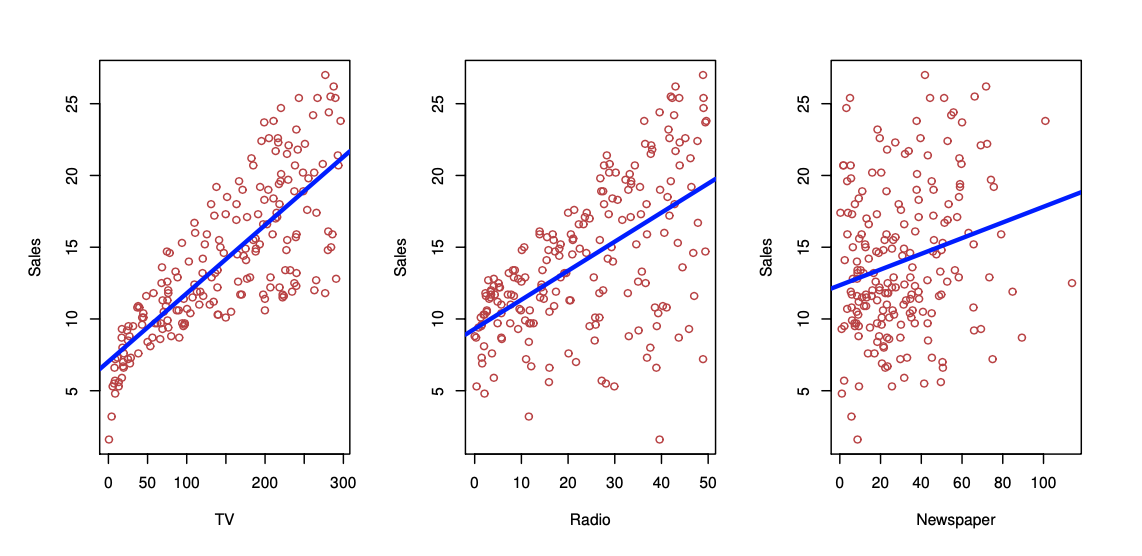## Linear Regression

• linear regression is a useful tool for predicting a quantitative response

• Serves as a good jumping-off point for newer approaches because many fancy statistical learning approaches can be seen as generalizations or extensions of linear regression

## Linear Regression and Advertising Data• Consider the advertising data shown above

• Questions that we might ask:

• Is there a relationship between advertising budget and sales?

• How strong is the relationship between advertising budget and sales?

• Which media contribute to sales?

• How accurately can we predict future sales?

• Is the relationship linear?

• Is there synergy among the advertising media?

• How could we answer these questions with linear regression?

## Simple Linear Regression

• A very straightforward approach for predicting a quantitative response $$Y$$ on the basis of a single predictor variable $$X$$

• The primary assumption that we make is that there is an approximately linear relationship between $$X$$ and $$Y$$

• We assume a model: $$Y = B_0 + B_1X + \epsilon$$

• where $$B_0$$ and $$B_1$$ are two unknown constants that represent the intercept and slope, also known as coefficients or parameters, and $$\epsilon$$ is the error term

• We may describe the above equation by saying that we are regressing $$Y$$ on $$X$$ (or $$Y$$ onto $$X$$)

• Once we have utilized our training data to produce estimates $$\hat{B_0}$$ and $$\hat{B_1}$$ for the model coefficients, we could predict future sales using: $$\hat{y} = \hat{B_0} + \hat{B_1}x$$

• where $$\hat{y}$$ indicates a prediction of $$Y$$ on the basis of $$X = x$$

• For our notation, a “hat” symbol denotes an estimated value

## Estimation of the Parameters by Least Squares

• Our goal is to obtain coefficient estimates $$\hat{B_0}$$ and $$\hat{B_1}$$ such that our linear model fits the available data well

• We want to find an intercept $$\hat{B_0}$$ and a slope $$\hat{B_1}$$ such that the resulting line is as close as possible to the $$n$$ data points

• There are a number of ways of measuring closeness. However, by far the most common approach involves minimizing the least squares criterion

• Let $$\hat{y} = \hat{B_0} + \hat{B_1}x$$ be the prediction for $$Y$$ based on the $$i$$th value of $$X$$

• Then $$e_i = y_i - \hat{y_i}$$ represent the $$i$$th residual

• The residual represents the difference between the $$i$$th observe response value and the $$i$$th response value that is predicted by our linear model

• We define the residual sum of squares (RSS) as: $$RSS = e_1^2 + e_2^2 + ... + e_n^2$$

• Or equivalently: $$RSS = (y_1-\hat{B_0}-\hat{B_1x_1})^2+(y_2-\hat{B_0}-\hat{B_1x_2})^2+...+(y_n-\hat{B_0}-\hat{B_1x_n})^2$$

• The least squares approach chooses the $$\hat{B_0}$$ and $$\hat{B_1}$$ that minimizes the RSS

• Let’s examine this at work by examining the advertising data